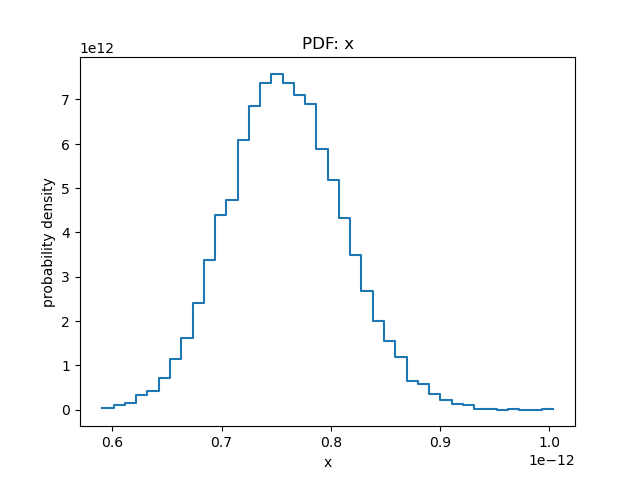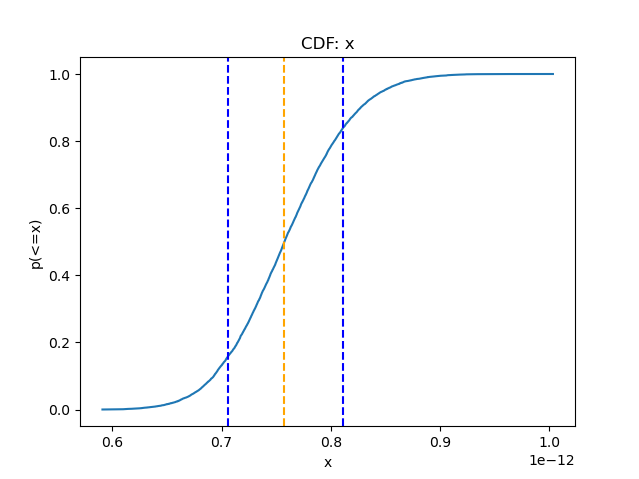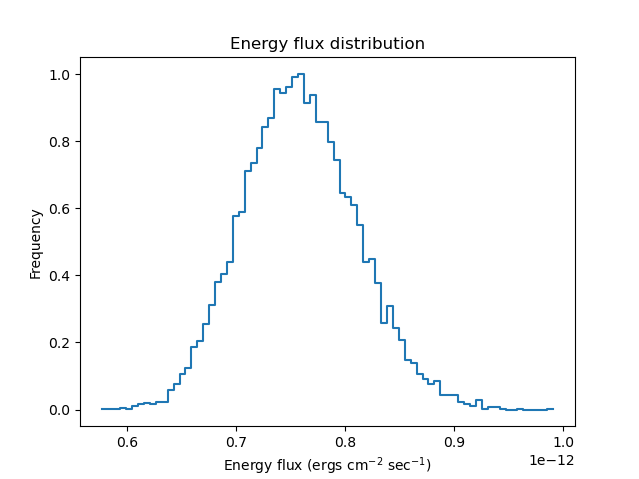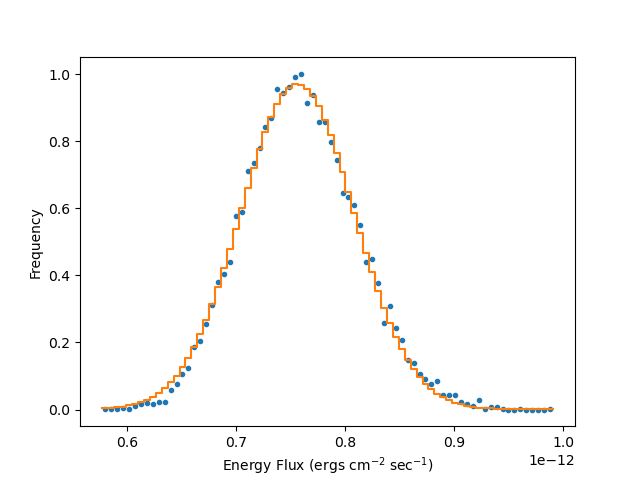# Calculating Uncertainties by Simulating Flux Distributions## Overview

#### Synopsis:

This thread demonstrates a method of determining flux uncertainties by sampling the parameter values using an uncorrelated, normal distribution.

Use this thread if you are interested in estimating the uncertainties in your modeled fluxes based on unthawed parameters.

Last Update: 6 Dec 2022 - Updated for CIAO 4.15; no content change.

## Introduction to the Flux Error Functions

Sherpa includes functions for creating and plotting flux probability distributions of Sherpa models—that is, the probability distribution of flux values that accounts for uncertainties in the model parameter values.

All thawed model parameters are sampled from the Gaussian distribution, where the mean is set as the best-fit parameter value and the variance is determined by the diagonal elements of the covariance matrix. The univariate Gaussian is assumed by default; treating each parameter independently. If there are correlations between parameters, then the multivariate Gaussian is available, using the off-diagonal elements of the covariance matrix. The flux is calculated for each sampled set of the parameters and recorded. The histogram of the simulated flux values gives the flux probability distribution for the assumed model.

The Sherpa flux error functions include the following:

## Getting Started

Please follow the Obtaining Data Used in Sherpa thread. In this example, we use the spectral data products for 3C 273, located in the pha_intro sub-directory.

## Fitting a Model to the Spectrum

We begin by loading the source spectrum, background, ARF and RMF with load_pha; filtering the data to model the 0.5-7.0 keV energy range; and subtracting the background.

sherpa> load_pha("3c273.pi")
sherpa> notice(0.5,7.0)
dataset 1: 0.00146:14.9504 -> 0.4672:9.8696 Energy (keV)

sherpa> subtract()


Choosing χ2 statistics, with variance calculated from the data, we fit an absorbed power-law model to the data using a neutral hydrogen column density of 0.07×1022 atoms/cm2.

sherpa> set_stat("chi2datavar")
sherpa> set_source(xsphabs.abs1*xspowerlaw.p1)
sherpa> abs1.nh = 0.07
sherpa> freeze(abs1)
sherpa> print(get_source())
(xsphabs.abs1 * xspowerlaw.pl)
Param        Type          Value          Min          Max      Units
-----        ----          -----          ---          ---      -----
abs1.nH      frozen         0.07            0        1e+06 10^22 atoms / cm^2
p1.PhoIndex  thawed            1           -3           10
p1.norm      thawed            1            0        1e+24

sherpa> fit()
Dataset               = 1
Method                = levmar
Statistic             = chi2datavar
Initial fit statistic = 1.2744e+11
Final fit statistic   = 35.9809 at function evaluation 22
Data points           = 42
Degrees of freedom    = 40
Probability [Q-value] = 0.651765
Reduced statistic     = 0.899521
Change in statistic   = 1.2744e+11
p1.PhoIndex    2.09882      +/- 0.061386
p1.norm        0.000214303  +/- 1.15866e-05


Note that it is possible to do freeze(abs1.nh) instead of freeze(abs1), which is useful when you only want to freeze a subset of the parameters of the component.

## Estimating Uncertainties by Simulating the Flux Distribution

We now simulate the energy flux between 0.5-7.0 keV one-hundred times, using sample_energy_flux, which returns one or more samples of the model flux, accounting for errors in the model parameters. The simulations choose parameters assuming their Gaussian distributions, with the mean set to the best-fit values and the variance given by the covariance matrix for all thawed parameters; the flux is then calculated for these parameters. The results will contain one-hundred realizations of the model and one-hundred values of the energy flux.

sherpa> flux100 = sample_energy_flux(0.5, 7, num=100)


Similarly, we may do this with the photon flux distribution using sample_photon_flux. Examining the structure of the array produced by sample_energy_flux:

sherpa> flux100.shape
(100, 4)

sherpa> print(flux100[0:5,:])
[[7.90478230e-13 2.14793376e+00 2.30945847e-04 0.00000000e+00]
[7.81851673e-13 2.01057247e+00 2.08004292e-04 0.00000000e+00]
[7.71279115e-13 2.11755807e+00 2.20911418e-04 0.00000000e+00]
[7.20391084e-13 2.10491727e+00 2.04609889e-04 0.00000000e+00]
[8.30436313e-13 2.04014101e+00 2.25622373e-04 0.00000000e+00]]


where we have used NumPy indexing and slicing to view a subset of the data.

### Determining Statistical Values from the Sample of Flux Values

The attributes of the array are the values of flux, photon spectral index, normalization factor, and a flag column indicating if the parameters were clipped (1) or not (0), respectively. The clipping flag was introduced in CIAO 4.13 to indicate whether or not the parameter value falls on the parameter limits. We can operate on these arrays to determine additional statistical values using the built-in NumPy functions, numpy.mean, numpy.median, and numpy.std from the distribution determined by the flux simulation.

Python arrays are called on as [row,column], so we can examine only the flux elements of the flux100 array by doing:

sherpa> print(flux100[:,0])
[7.90478230e-13 7.81851673e-13 7.71279115e-13 7.20391084e-13
8.30436313e-13 6.99769586e-13 7.94232936e-13 6.97348523e-13
7.24854578e-13 7.87956562e-13 6.99324624e-13 7.80001547e-13
…
7.64157350e-13 7.21386453e-13 7.81280986e-13 7.66305892e-13
7.50408359e-13 7.83207311e-13 7.23071470e-13 7.48506546e-13
8.50902661e-13 8.56211502e-13 8.06796644e-13 7.42217963e-13]


and using the NumPy functions to determine the mean, median, and standard deviation of the sample of fluxes; in units of ergs/cm2/sec, we find:

sherpa> fluxes = flux100[:,0]

sherpa> np.mean(fluxes)
7.578636610539687e-13

sherpa> np.median(fluxes)
7.592345840008671e-13

sherpa> np.std(fluxes)
5.383019543868312e-14


### Determining Quantile Values of the Flux Sample

By estimating the number of points in the array—which is known from the number of simulations performed using the information from the len and NumPy sort functions—the quantiles for this sample may be obtained.

First, we sort the fluxes into an 1D array in ascending order:

sherpa> sf = np.sort(fluxes)

sherpa> print(sf[:10]) # check sorting by printing the first 10 elements
[  6.53277377e-13   6.57256162e-13   6.59786070e-13   6.64675896e-13
6.66676371e-13   6.68632405e-13   6.71511228e-13   6.73479647e-13
6.79610177e-13   6.81313606e-13]


and then get the value of the flux for the 95% quantile and 50% quantile, which corresponds to the median. Since we ran one-hundred simulations, we know that we are looking for the 95th and 50th elements of the array. But more generally, the quantiles can be determined using the length function, len, to determine the number of elements in the column.

sherpa> sf[int(0.95*len(fluxes)-1)]
8.399304082068919e-13

sherpa> sf[int(0.5*len(fluxes)-1)]
7.592086660490975e-13


Remembering that the array indicies begin at zero, we subtract one from the quantile value.

### Probability Density and Cumulative Distributions

The plotting functions plot_pdf and plot_cdf provide a simple way to display the probability density and cumulative distribution for the flux arrays.

We start with generating a large number of simulations and plotting the PDF and CDF functions of the flux arrays stored in the first element of the array, numbered 0. We also use get_cdf_plot to obtain the characteristic values for the distribution.

sherpa> flux10000 = sample_energy_flux(0.5, 7, num=10000)

sherpa> flux10000.shape
(10000, 4)

sherpa> f = flux10000[:,0]

sherpa> plot_pdf(f, bins=40)

sherpa> plot_cdf(f)

sherpa> cplot = get_cdf_plot()

sherpa> cplot.median
7.573272785556908e-13

sherpa> cplot.lower
7.069957077924039e-13

sherpa> cplot.upper
8.101846158068927e-13


which produces the PDF (Figure 1) and CDF (Figure 2) plots shown below.

### Figure 1: Flux Probability Density### Figure 2: Flux Cumulative Distribution## Creating a Histogram of a Flux Probability Distribution

We can visualize the flux distribution with a histogram created by the get_energy_flux_hist function and check whether the distribution is Gaussian or another shape. We simulate the flux distribution between 0.5-7.0 keV ten-thousand times, to produce good statistics. We use the function get_energy_flux_hist, which produces a data object that contains all the information about the simulated sample of parameters and a histogram, normalized to unity, representing the flux probability distribution. By default, get_energy_flux_hist creates a histogram with 75 bins; however, the optional parameter, bins, may be included to change the binning.

sherpa> hist10000 = get_energy_flux_hist(0.5, 7, num=10000)


Similarly, this may be done in photon units with get_photon_flux_hist. To learn more about the data product produced by get_photon_flux_hist, enter 'ahelp "get_photon_flux_hist"' on the Sherpa command-line.

We may display the flux probability distribution histogram determined by get_energy_flux_hist using plot_energy_flux, which plots the calculated energy flux probability distribution—which is the flux distribution for the model component accounting for the errors on the model parameters (Figure 3).

sherpa> plot_energy_flux(0.5, 7, recalc=False)


### Figure 3: Histogram of the Flux Distribution### Figure 3: Histogram of the Flux Distribution

This is a histogram of the energy flux probability distribution, plotted using plot_energy_flux, which is determined by get_energy_flux_hist.

### Determining the Uncertainties from the Flux Distributions

Taking the histogram data array, we replot the histogram as a scatter plot to check the probability distribution with a Gaussian function, fitting the Gaussian to the histogram with least-squares statistics.

sherpa> load_arrays(2, hist10000.xlo, hist10000.xhi, hist10000.y, Data1DInt)
sherpa> plot_data(2)
WARNING: The displayed errorbars have been supplied with the data or calculated using chi2xspecvar; the errors are not used in fits with chi2datavar
zeros or negative values found

sherpa> set_source(2, gauss1d.g0)
sherpa> g0.integrate = False
sherpa> guess(2, g0)
sherpa> set_par(g0.ampl, min=0, max=10, val=1)
sherpa> set_stat("leastsq")

sherpa> fit(2)
Dataset               = 2
Method                = levmar
Statistic             = leastsq
Initial fit statistic = 0.244015
Final fit statistic   = 0.0588359 at function evaluation 17
Data points           = 76
Degrees of freedom    = 73
Change in statistic   = 0.185179
g0.fwhm        1.23318e-13  +/- 3.89481e-14
g0.pos         7.54643e-13  +/- 2.02552e-14
g0.ampl        0.916171     +/- 0


We use load_arrays to load the histogram flux probability distribution into dataset ID=2, where hist10000.xlo and hist10000.xhi are the low and high bins in the histogram and hist10000.y is the probability of getting the flux within the bin.

Plotting this fit, we can see the good agreement between the Gaussian distribution and flux probability distribution (Figure 4).

sherpa> plot_fit(2, yerrorbars=False)
sherpa> limits(Y_AXIS, -0.05, 1.05)
sherpa> plt.ylabel("Frequency")
sherpa> plt.xlabel(r"Energy Flux (ergs cm$^{-2}$ sec$^{-1}$)")


### Figure 4: Gaussian Fitted Flux Distribution### Figure 4: Gaussian Fitted Flux Distribution

The energy flux probability distribution replotted as a scatter-plot with a Gaussian function fitted to the data (red).

We can now determine the uncertainty in the modeled flux by calculating the standard deviation, instead of the FWHM of the Gaussian, using the relationship: $$\mathrm{FWHM} = \sigma \sqrt{8 \ln{2}} \approx 2.35482 \sigma$$.

sherpa> fac0 = np.sqrt(8 * np.log(2))
sherpa> sig = g0.fwhm.val/fac0

sherpa> sig
5.236815148165619e-14


Since we have generated two distributions of fluxes, we can examine the results of the mean of the flux100 sample and compare it with the mean position of the Gaussian fit to the histogram, g0.pos. We see that the two mean values, 〈flux100〉 ≅ 7.579×10-13 ergs cm-2 s-1 and 〈hist10000〉 ≅ 7.594×10-13 ergs cm-2 s-1, are approximately the same. The standard deviations, similarly, are equivalent to each other, σflux100 ≅ 5.383×10-14 ergs cm-2 s-1 and σhist10000 ≅ 5.237×10-14 ergs cm-2 s-1. This is expected for normal distributions, as was simulated; however, if the flux distribution were different (e.g. skewed-, bi-modal-, or κ-distributions), then the calculated mean and σ using NumPy may be very different. Visualization of the distributions using the histogram is a useful sanity check.

We can write the array to an ASCII file using the save_arrays function, including column headers:

sherpa> save_arrays("hist10000.dat", [hist10000.xlo,hist10000.xhi,hist10000.y], fields=["xlo","xhi","y"])


and reload the simulation results into another session with the load_ascii function:

sherpa> load_ascii("hist10000.dat", ncols=3, dstype=Data1DInt)


for future analysis.

## Scripting It

The file, fit.py , performs the primary commands used above. It can be executed by typing exec(open("fit.py").read()) on the Sherpa command line. The Sherpa script command may be used to save everything typed on the command line in a Sherpa session:

sherpa> script(filename="sherpa.log", clobber=False)


(Note that restoring a Sherpa session from such a file could be problematic since it may include syntax errors, unwanted fitting trials, et cetera.)

## History

 04 Aug 2009 Updated for S-Lang users to take advantage of the new "ciao_utils" scripts module. 30 Apr 2009 New for CIAO 4.1 02 Dec 2009 Updated for CIAO 4.2: the sherpa_contrib.flux_dist routines are now available from Sherpa; save_arrays is now available. 13 Jul 2010 updated for CIAO 4.2 Sherpa v2: removal of S-Lang version of thread. 15 Dec 2011 updated for CIAO 4.4: plot_pdf and plot_cdf, and associated functions, are now available 13 Dec 2012 updated for CIAO 4.5: the sample_flux function is now available 10 Dec 2013 Updated for CIAO 4.6. 10 Dec 2014 Updated for CIAO 4.7; no content change. 12 Jul 2018 Updated for CIAO 4.10; no content change. 11 Dec 2018 Updated for CIAO 4.11; revised screen output 22 Mar 2022 Updated for CIAO 4.14; figures replaced with Matplotlib plots. 06 Dec 2022 Updated for CIAO 4.15; no content change.